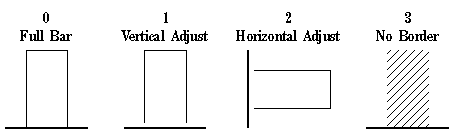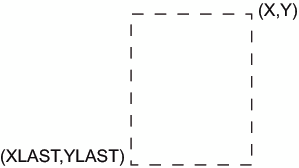# BAR Function

Draws a rectangle whose lower left corner is defined by the internal variables (XLAST, YLAST) and whose upper right corner is defined by the specified X, Y variable pair. You can define the color of the fill, the fill pattern, and the edge lines to be drawn.

## Syntax

FUNCTION='BAR';

### Associated Variables

COLOR='color'
specifies the color of either the interior of the bar or the outline of the bar. Color can be any SAS/GRAPH color name. The part of the bar affected depends on the value of the STYLE variable. If STYLE specifies a pattern or fill, the COLOR variable determines the color of the interior. If STYLE specifies an empty pattern, the COLOR variable determines the color of the outline of the bar.
GROUP=group-value
MIDPOINT=midpoint-value
SUBGROUP=subgroup-value
specify coordinates for HBAR and VBAR charts from the GCHART procedure. Use these variables only with the data coordinate systems 1, 2, 7, and 8.
specifies the text that defines the link for drill-down.
LINE=0...3
specifies the direction in which to adjust the outline of the bar. Use LINE values 1 and 2 to offset a particular bar from an axis or adjoining area. The following figure illustrates LINE values.
LINE Values for BarsDefault:1
SIZE=thickness
specifies a line thickness for the rectangle
STYLE='fill-pattern'
specifies the pattern that fills the bar. Fill-pattern can be the following bar and block patterns:
SOLID
S
a solid fill.
EMPTY
E
an empty fill.
style<density>
• style can be R | X | L
• density can be 1...5
Note:Java and ActiveX support only SOLID and EMPTY. EMPTY is the default if any other value is used.
WHEN='B' | 'A'
specifies when to draw the bar in relation to other procedure output. See
X=horizontal-coordinate
Y=vertical-coordinate
Z=depth-coordinate
XC='character-type-horizontal-coordinate'
YC='character-type-vertical-coordinate'
define the upper right corner of a bar (rectangle) whose lower left corner is (XLAST,YLAST). Use the Z variable only when you are annotating output from the G3D procedure. Points Used to Construct a Bar illustrates the use of these coordinates.
XSYS='coordinate-system'
specifies the coordinate system for the X or XC variable. The XC variable can be used only with XSYS='2'. See XSYS Variable for an explanation of coordinate-system.
YSYS='coordinate-system'
specifies the coordinate system for Y or YC variable. The YC variable can be used with YSYS='2' only. See YSYS Variable for an explanation of coordinate-system.
ZSYS='coordinate-system'
specifies the coordinate system for the Z variable. See ZSYS Variable for an explanation of coordinate-system.

## Details

Points Used to Construct a Bar shows how the XLAST, YLAST, and X, Y variables define the diagonal corners of the bar. With character data, the XC and YC variables are used in place of the X and Y variables. The values of the XLAST and YLAST variables are usually initialized with a MOVE function or another function that updates the XLAST and YLAST pair. When the XC variable is used, set XSYS='2'. When the YC variable is used, set YSYS='2'.
Points Used to Construct a Bar